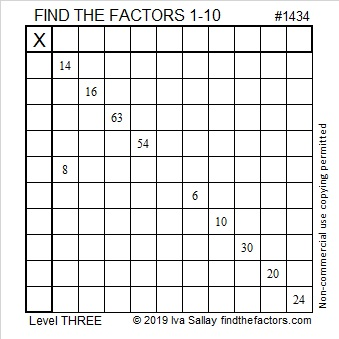# 1434 and Level 3

If you know the common factors of 14 and 8, then you will have an excellent start to solve this puzzle. Once you place the factors of 18 and 8 in their proper places, just work down the puzzle row by row until all the factors from 1 to 10 are found.Print the puzzles or type the solution in this excel file:  10 Factors 1432-1442

Now I’ll share some facts about the puzzle number, 1434:

• 1434 is a composite number.
• Prime factorization: 1434 = 2 × 3 × 239.
• 1434 has no exponents greater than 1 in its prime factorization, so √1434 cannot be simplified.
• The exponents in the prime factorization are 1, 1, and 1. Adding one to each exponent and multiplying we get (1 + 1)(1 + 1)(1 + 1) = 2 × 2 × 2 = 8. Therefore 1434 has exactly 8 factors.
• The factors of 1434 are outlined with their factor pair partners in the graphic below.1434 is 234 in BASE 26 because
2(26²) + 3(26¹) + 4(26º) = 1434